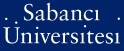# Viscosity Solutions: An Introduction to the basics of the theory

Baydil, Banu (2002) Viscosity Solutions: An Introduction to the basics of the theory. [Thesis]PDF - Registered users only - Requires a PDF viewer such as GSview, Xpdf or Adobe Acrobat Reader889Kb

## Abstract

In this work, concepts that appear in the basic theory of viscosity solutions theory is surveyed. Structures of sub and super di.erentials and sub and super semijets, and concepts of generalized second derivative, generalized 'maximum principle' and generalized 'comparison principle' are studied. Basic properties of semiconvex functions and sup (Jensen's) convolutions are presented. Existence and uniqueness of solutions of the Dirichlet Problem for first and second order nonlinear elliptic partial differential equations is studied.

Item Type: Thesis Viscosity solutions. -- Nonlinear elliptic partial differential equations. -- Maximum principles. -- Comparison theorems. -- Perron's method Q Science > QA Mathematics 8140 IC-Cataloging 18 Apr 2008 11:19 27 Dec 2008 11:18

Repository Staff Only: item control page